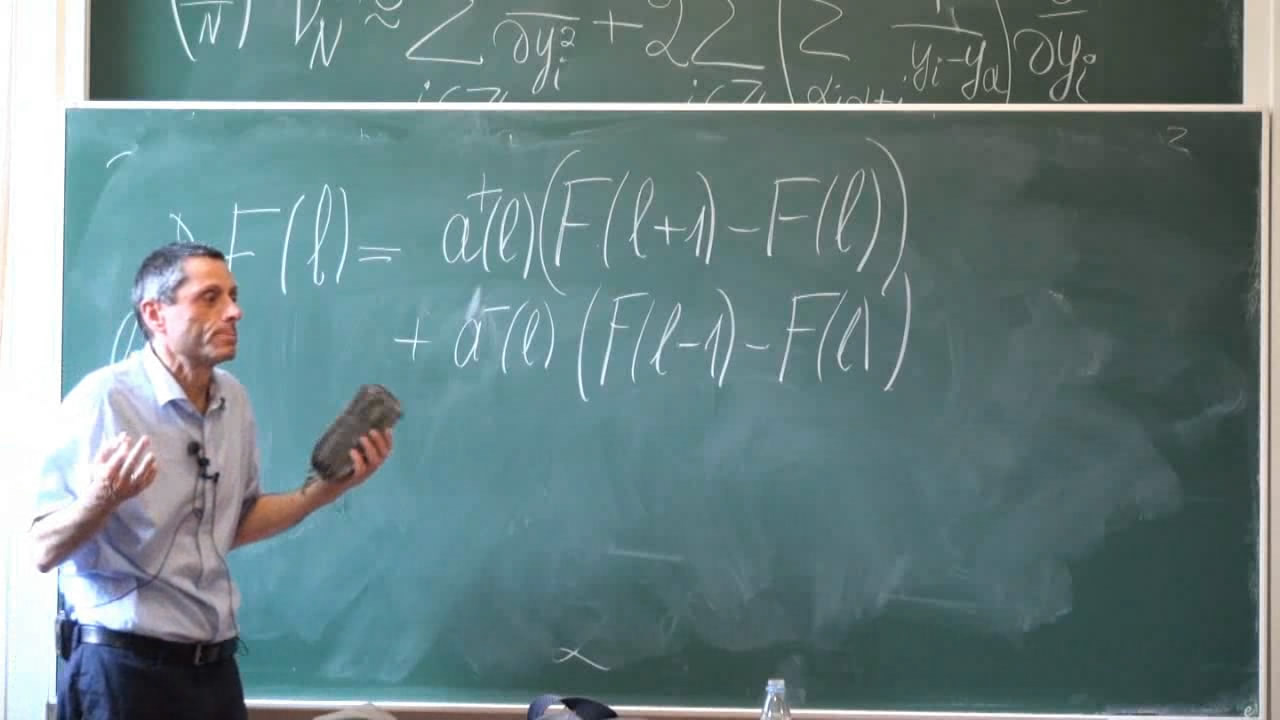Non-colliding processes with infinitely many particles

Курс Хит
Предмет:Models of Markov dynamics for N interacting non-colliding particles (N=1,2,...) have been studied in the Random Matrix literature since Dyson's paper (1962) on the matrix-valued Brownian motion. However, extension of the theory to the case of infinitely many particles presents substantial difficulties. For instance, one wants to take an appropriate large-N limit, but it is difficult to justify it, to prove that the Markov property is not destroyed, or to make sense of a formal expression for the hypothetical infinite-particle generator. I will describe a new and relatively elementary method of constructing models of infinite-dimensional Markov dynamics based on some ideas from representation theory of infinite-dimensional groups.

Курс прочитан в рамках St. Petersburg School in Probability and Statistical Physics.

Информация о курсе

3